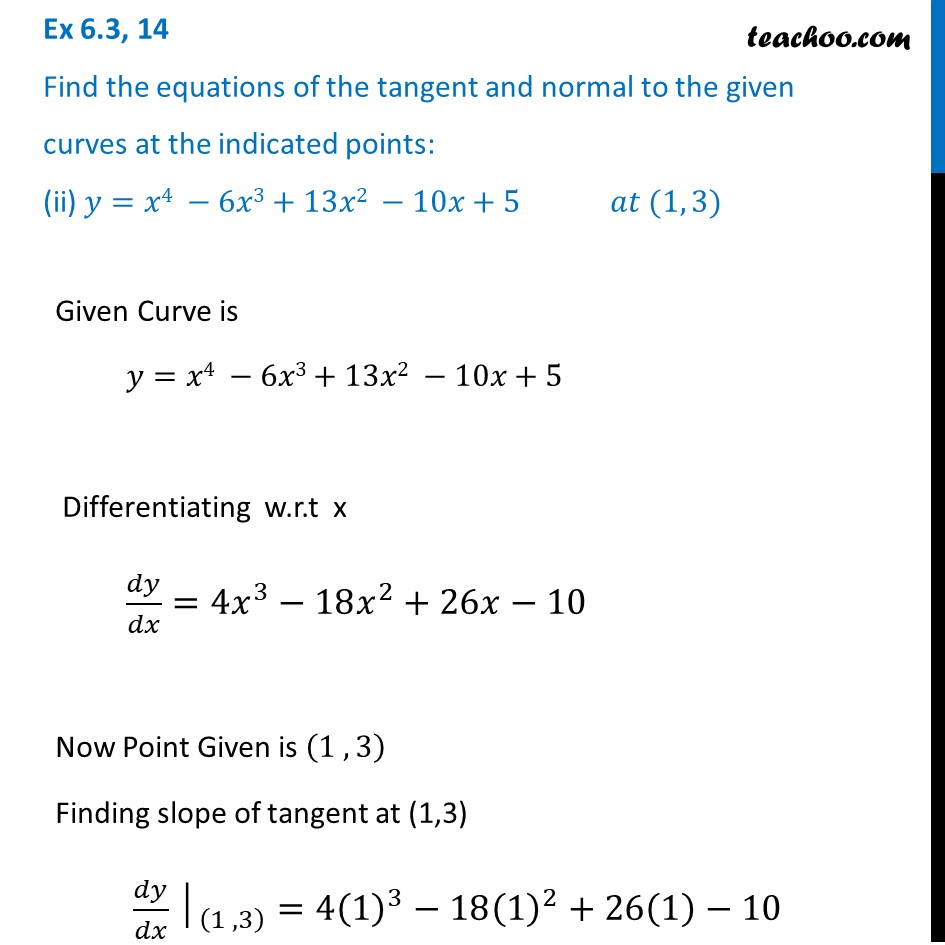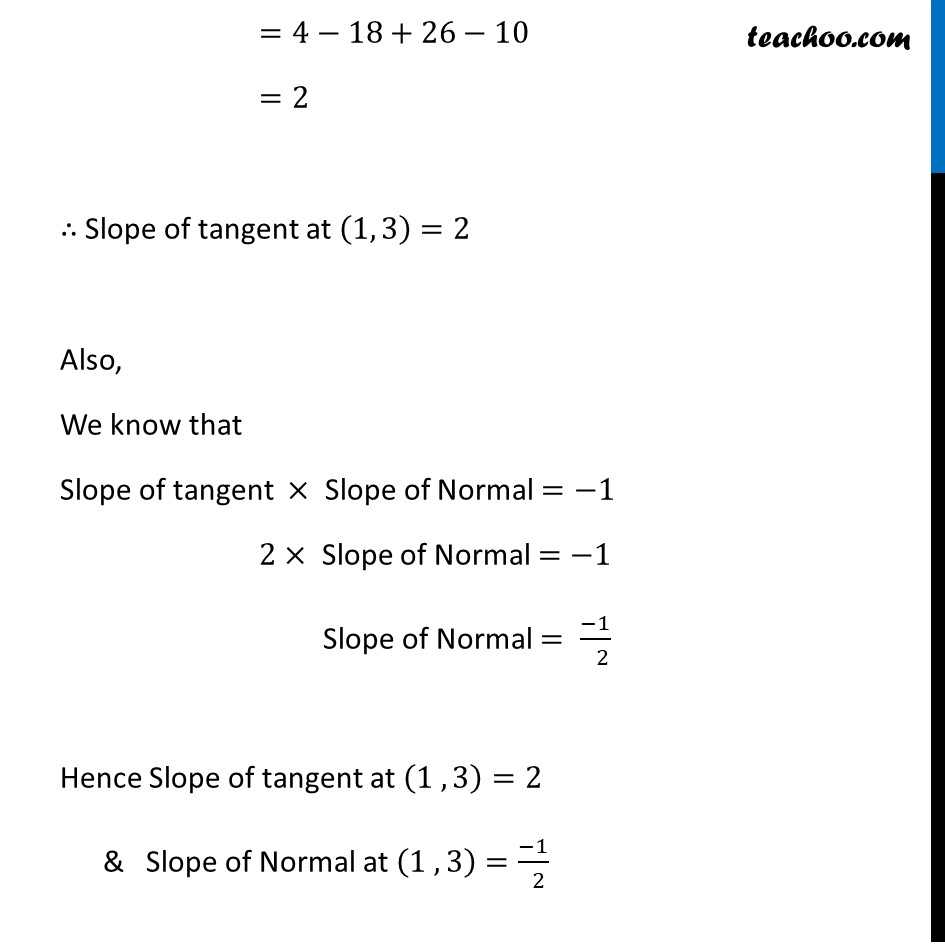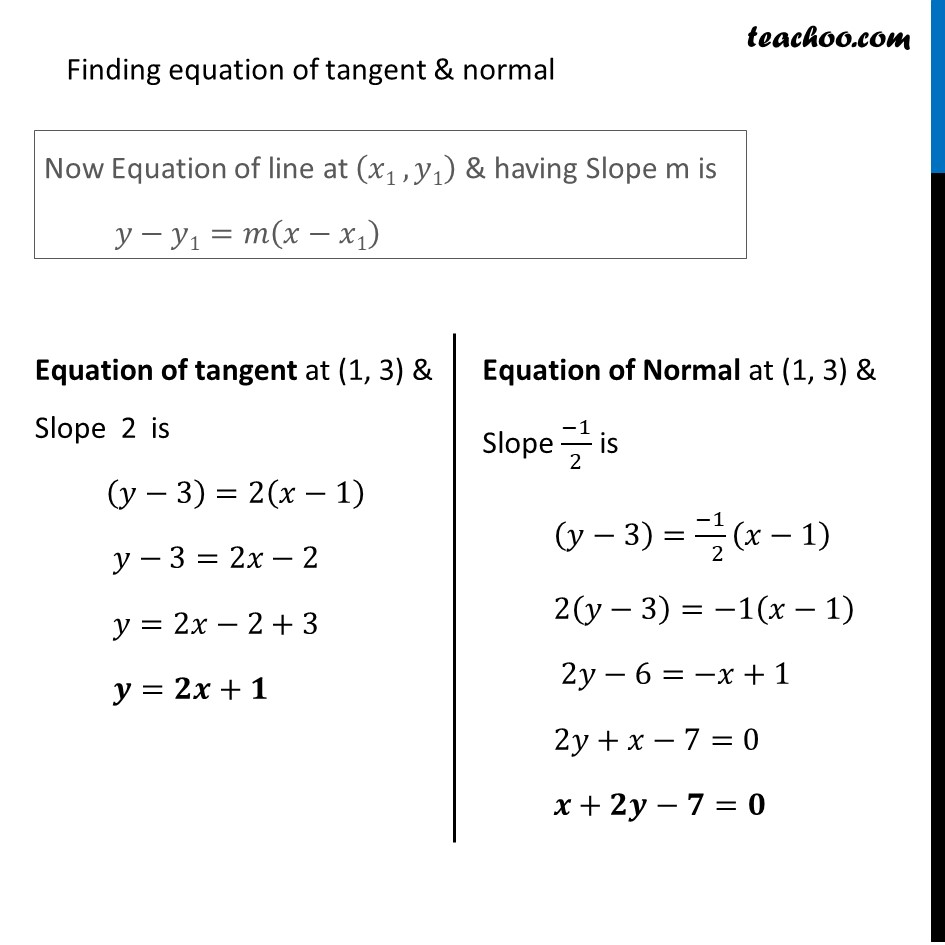Tangents and Normals (using Differentiation)

Chapter 6 Class 12 Application of Derivatives
Serial order wiseLearn in your speed, with individual attention - Teachoo Maths 1-on-1 Class

### Transcript

Question 14 Find the equations of the tangent and normal to the given curves at the indicated points: (ii) 𝑦=𝑥4 −6𝑥3+13𝑥2 −10𝑥+5 𝑎𝑡 (1, 3) Given Curve is 𝑦=𝑥4 −6𝑥3+13𝑥2 −10𝑥+5 Differentiating w.r.t x 𝑑𝑦/𝑑𝑥=4𝑥^3−18𝑥^2+26𝑥−10 Now Point Given is (1 , 3) Finding slope of tangent at (1,3) 〖𝑑𝑦/𝑑𝑥│〗_((1 , 3) )=4(1)^3−18(1)^2+26(1)−10 =4−18+26−10 =2 ∴ Slope of tangent at (1, 3) =2 Also, We know that Slope of tangent × Slope of Normal =−1 2× Slope of Normal =−1 Slope of Normal = (−1)/( 2) Hence Slope of tangent at (1 , 3)=2 & Slope of Normal at (1 , 3)=(−1)/( 2) Finding equation of tangent & normal Now Equation of line at (𝑥1 , 𝑦1) & having Slope m is 𝑦−𝑦1=𝑚(𝑥−𝑥1) Equation of tangent at (1, 3) & Slope 2 is (𝑦−3)=2(𝑥−1) 𝑦−3=2𝑥−2 𝑦=2𝑥−2+3 𝒚=𝟐𝒙+𝟏 Equation of Normal at (1, 3) & Slope (−1)/2 is (𝑦−3)=(−1)/( 2) (𝑥−1) 2(𝑦−3)=−1(𝑥−1) 2𝑦−6=−𝑥+1 2𝑦+𝑥−7=0 𝒙+𝟐𝒚−𝟕=𝟎